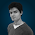## Monday, 7 May 2012

### Implausible Extrapolation?

No, I'm not about to go off on a rant about the flawed logic involved in deriving a macroevolutionary hypothesis from the observable processes of microevolution.  I'm going to answer Tim's obscure mathematical query, Question 4: If a=9, b=30 and c=25, what does x equal?

Part of me just wants to say 42.

But despite the inherent sci-fi coolness of that answer, it might be cheating.

Also, I have a cleverer theory, and it goes something like this:
• For any given string of numbers, however random, you should be able to construct a triangle of differences.
• First off, the numbers themselves are 9, 30 and 25.
• Therefore, the differences between consecutive numbers in the sequence are 21 and -5.
• Therefore, the difference between the differences is -26.
• So we now have a triangle, like so:
9          30          25
21          -5
-26
• If each row is extrapolated towards the right, assuming the difference between the differences is a constant -26, we get something like this:
9          30          25         -6         -63         -146 ...
21          -5          -31          -57          -83 ...
-26          -26          -26          -26 ...
• Assuming a to be the first number, b to be the second and c to be the third, it seems logical to say that x must be the 24th, since it is the 24th letter of the alphabet.
• x, i.e. the 24th value in a sequence which begins with 9 and has a difference between differences of -26
• And if this line of logic is followed far enough, you can determine the value of the nth number in the sequence, for any n.
• I think the formula works out as...
• *insert a longish pause while I try and fail to boil all those numbers down to a simple equation*
• Well, what I've got so far is " n(x) = ( n(2) - n(1) ) + ", which isn't even a complete equation, and I'm pretty sure it would still be wrong even if I'd bothered to finish it.
• Stuff this for a lark; I'm going to fire up Excel and get it to calculate x for me!
• My helpful spreadsheet program tells me that the 24th number in this sequence, i.e. x, is -6086.
• x must therefore be -6086.  Because Microsoft Excel 2007 says so.

Okay, that was arcane.

Perhaps I should have settled for '42'?

Compulsory Stats:
• Last 10 for 17 status: neck and neck, each with 2 down, 8 to go.
• Latest book read: still The Kink and I
• Latest film/TV watched: Logan's Run - absolutely dreadful film; don't bother watching it!
• Latest music listened to: something by Yanni
• Latest edible item eaten: Smarties, and cinnamon hot chocolate
• Programs and web pages currently running: Microsoft Office Outlook, Word and Excel 2007, Firefox (tabs: Blogspot Dashboard; Blogspot Create Post; MatNav 6.1)
• Webcomics posted today: Cylinder and Miserable Episode 1473

- The Colclough

1.I must confess that your answer is far more interesting than the one I was thinking of, and you found it in a far more interesting way. So the points go to you. However, the actual value of x that I had been thinking of was -5/3. A classic formula that uses the terms a, b, c, and x is a quadratic formula. A quadratic formula is in the form ax^2+bx+c=0, thus is in instance the formula is 9x^2+30x+25=0. To find if x has more than one solution, use the quadratic formula: -b/2a +or- sqr root of b^2-4ac. Which happens to work out as 30/18 +or- sqr root of 0/18. That proves there is only one answer for x. Factorising the equation give (3x+5)^2=0. Divide both sides by themselves, and you get 3x+5=0. That can be rearranged as 3x=-5. Divide by 3 to get x on it's own... x=-5/3.

1.i did know that stuff once. but i never enjoyed it at the time and i've been happily forgetting all about it ever since finishing my maths GCSE, which was... *counts slowly* about 8 years ago, so i'm not surprised i didn't cotton on to the quadratic possibilities.

2.My Brain Hurts.

1.condolences. mine didn't feel to great by the time i'd finished writing this post either.

3.It took almost 8 attempts to post that comment, is there an easier way?

1.not that i'm aware of.